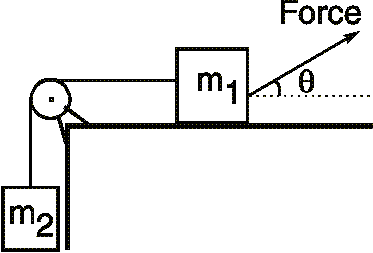# Forces in a Pulley System

## Homework StatementMass m1=26.1 kg is on a horizontal surface, connected to mass m2= 5.50 kg by a light string as shown. The pulley has negligible mass and no friction. A force of 197.7 N acts on m1 at an angle of 33.3 deg.
The coefficient of kinetic friction between m1 and the surface is 0.233. Determine the upward acceleration of m2.
2.44 m/s^2

## Homework Equations

a1 = a2

T1 = T2

T1 = Fcos(theta) - Frictionforce

Friction force = uk(Normal Force)

Normal Force = weight - Fsin(theta)

## The Attempt at a Solution

I'm actually looking at this problem again since I first did a week ago and I don't have the sheet I worked it out on with me right now, but using the equations I wrote above keeping in mind that T1=T2 I pretty much worked the whole thing out, however when I entered it into the online homework system I got it wrong no matter what I did I just couldn't get it. Can someone help me out with what I'm doing wrong? By the way I know what the answer is, but I just no how you would go about getting to it, I step-by-step run down through the problem would be helpful.

Doc Al
Mentor
a1 = a2

T1 = T2
These are OK.

T1 = Fcos(theta) - Frictionforce
This assumes equilibrium, which is not the case here: the masses are accelerating. For each mass, apply Newton's 2nd law. You'll get two equations which you can solve together.

Friction force = uk(Normal Force)

Normal Force = weight - Fsin(theta)
OK.

Is the weight both masses added together? I have this same question with different numbers/masses.

is friction force the same as mass*acceleration = u*normal force?

Doc Al
Mentor
Is the weight both masses added together? I have this same question with different numbers/masses.
No. Why would you add the weights together?
is friction force the same as mass*acceleration = u*normal force?
The friction force will equal μ*N. (But don't set it equal to mass*acceleration--other forces are acting.)